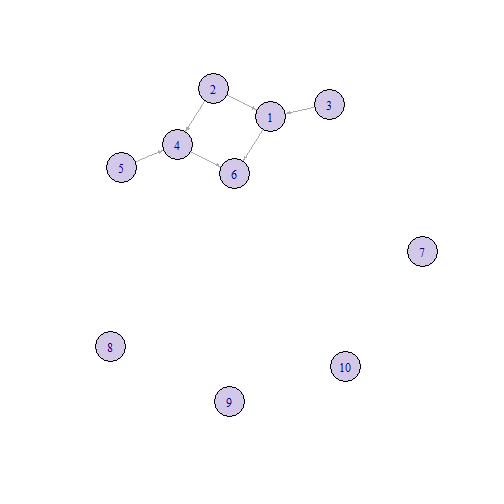title: “BiCausality: Binary Causality Inference Framework” author: “ C. Amornbunchornvej” date: “2022-08-19” output: rmarkdown::html_vignette vignette: > %\VignetteIndexEntry{BiCausality_demo} %\VignetteEngine{knitr::knitr}

## Example: Inferred binary causal graph from simulation

In the first step, we generate a simulation dataset as an input.

``````seedN<-2022

n<-200 # 200 individuals
d<-10 # 10 variables
mat<-matrix(nrow=n,ncol=d) # the input of framework

#Simulate binary data from binomial distribution where the probability of value being 1 is 0.5.
for(i in seq(n))
{
set.seed(seedN+i)
mat[i,] <- rbinom(n=d, size=1, prob=0.5)
}

mat[,1]<-mat[,2] | mat[,3]  # 1 causes by 2 and 3
mat[,4] <-mat[,2] | mat[,5] # 4 causses by 2 and 5
mat[,6] <- mat[,1] | mat[,4] # 6 causes by 1 and 4
``````

We use the following function to infer whether X causes Y.

``````# Run the function
library(BiCausality)
resC<-BiCausality::CausalGraphInferMainFunc(mat = mat,CausalThs=0.1, nboot =50, IndpThs=0.05)
``````
``````## Inferring dependent graph
``````
``````## Removing confounder(s)
``````
``````## Inferring causal graph
``````

The result of the adjacency matrix of the directed causal graph is below:

``````resC\$CausalGRes\$Ehat
``````
``````##       [,1] [,2] [,3] [,4] [,5] [,6] [,7] [,8] [,9] [,10]
##  [1,]    0    0    0    0    0    1    0    0    0     0
##  [2,]    1    0    0    1    0    0    0    0    0     0
##  [3,]    1    0    0    0    0    0    0    0    0     0
##  [4,]    0    0    0    0    0    1    0    0    0     0
##  [5,]    0    0    0    1    0    0    0    0    0     0
##  [6,]    0    0    0    0    0    0    0    0    0     0
##  [7,]    0    0    0    0    0    0    0    0    0     0
##  [8,]    0    0    0    0    0    0    0    0    0     0
##  [9,]    0    0    0    0    0    0    0    0    0     0
## [10,]    0    0    0    0    0    0    0    0    0     0
``````

The value in the element EValHat[i,j] represents that i causes j if the value is not zero. For example, EValHat[2,1] = 1 implies node 2 causes node 1, which is correct since node 1 have nodes 2 and 3 as causal nodes.

The directed causal graph also can be plot using the code below.

``````library(igraph)
``````
``````##
## Attaching package: 'igraph'
``````
``````## The following objects are masked from 'package:stats':
##
##     decompose, spectrum
``````
``````## The following object is masked from 'package:base':
##
##     union
``````
``````net <- graph_from_adjacency_matrix(resC\$CausalGRes\$Ehat ,weighted = NULL)
plot(net, edge.arrow.size = 0.3, vertex.size =20 , vertex.color = '#D4C8E9',layout=layout_with_kk)
``````For the causal relation of variables 2 and 1, we can use the command below to see further information.

**Note that the odd difference between X and Y denoted oddDiff(X,Y) is define as |P (X = 1, Y = 1) P (X = 0, Y = 0) −P (X = 0, Y = 1) P (X = 1, Y = 0)|. If X is directly proportional to Y, then oddDiff(X,Y) is close to 1. If X is inverse of Y, then oddDiff(X,Y) is close to -1. If X and Y have no association, then oddDiff(X,Y) is close to zero.

``````resC\$CausalGRes\$causalInfo[['2,1']]
``````
``````## \$CDirConfValInv
##  2.5% 97.5%
##     1     1
##
## \$CDirConfInv
##      2.5%     97.5%
## 0.3152526 0.4386415
##
## \$CDirmean
##  0.371347
##
## \$testRes2
##
##  Wilcoxon signed rank test with continuity correction
##
## data:  abs(bCausalDirDist)
## V = 1275, p-value = 3.893e-10
## alternative hypothesis: true location is greater than 0.1
##
##
## \$testRes1
##
##  Wilcoxon signed rank test with continuity correction
##
## data:  abs(bSignDist)
## V = 1275, p-value = 3.889e-10
## alternative hypothesis: true location is greater than 0.05
##
##
## \$sign
##  1
##
## \$SignConfInv
##      2.5%     97.5%
## 0.0865425 0.1282719
##
## \$Signmean
##  0.1090915
``````

Below are the details of result explanation.

``````#This value represents the 95th percentile confidence interval of P(Y=1|X=1).
\$CDirConfValInv
2.5% 97.5%
1     1
#This value represents the 95th percentile confidence interval of |P(Y=1|X=1) - P(X=1|Y=1)|.
\$CDirConfInv
2.5%     97.5%
0.3217322 0.4534494

#This value represents the mean of |P(Y=1|X=1) - P(X=1|Y=1)|.
\$CDirmean
 0.3787904

#The test that has the null hypothesis that |P(Y=1|X=1) - P(X=1|Y=1)| below
#or equal the argument of parameter "CausalThs" and the alternative hypothesis
#is that |P(Y=1|X=1) - P(X=1|Y=1)| is greater than "CausalThs".
\$testRes2

Wilcoxon signed rank test with continuity correction

data:  abs(bCausalDirDist)
V = 1275, p-value = 3.893e-10
alternative hypothesis: true location is greater than 0.1

#The test that has the null hypothesis that |oddDiff(X,Y)| below
#or equal the argument of parameter "IndpThs" and the alternative hypothesis is
#that |oddDiff(X,Y)| is greater than "IndpThs".
\$testRes1

Wilcoxon signed rank test with continuity correction

data:  abs(bSignDist)
V = 1275, p-value = 3.894e-10
alternative hypothesis: true location is greater than 0.05

#If the test above rejects the null hypothesis with the significance threshold
#alpha (default alpha=0.05), then the value "sign=1", otherwise, it is zero.
\$sign
 1

#This value represents the 95th percentile confidence interval of oddDiff(X,Y)
\$SignConfInv
2.5%      97.5%
0.08670325 0.13693900

#This value represents the mean of oddDiff(X,Y)
\$Signmean
 0.1082242
``````